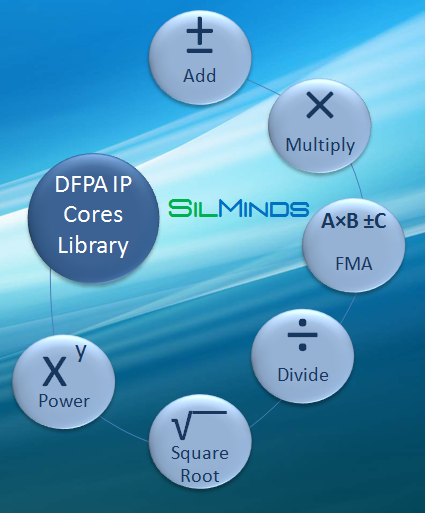# SilMinds

## DFP arithmetic librarary (DecLib)

DecLib is an extensible IP unit library that comprises all basic arithmetic and logic operations, format conversions,  as well as a number of transcendental functions. These units can be scaled up to deliver area-efficient higher throughput by means of internal core pipelining or multiplicity. DecLib is backed up by over a dozen refereed publications and eight pending U.S. patents. All units support 64 and 128 bit numbers (16 and 34 decimal digits, respectively) with either DPD (Densely Packed Decimal) or BID (Binary Integer Decimal) as required; in compliance with IEEE 754-2008 for computer arithmetic.

SilMinds DecLib has pioneered the following accomplishments and novel features:

• Energy savings consideration in DFP arithmetic IPs
• Implementation o a fused multiply-add function
• Implementation of a Newton-Raphson divider; over three times faster than previous similar designDecAcc (DFP accumulator)

DecMFU (DFP logic)

DecMult (DFP multiplier)